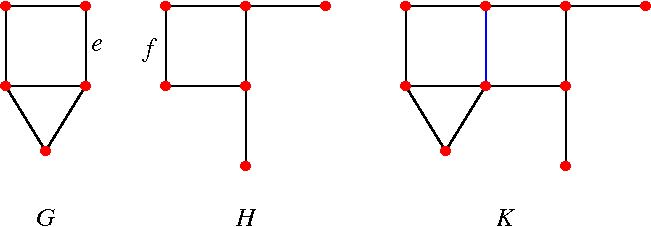18.314 | Fall 2014 | Undergraduate
Combinatorial Analysis
Assignments

## Problem Set 11

Most of the problems are assigned from the required textbook Bona, Miklos. A Walk Through Combinatorics: An Introduction to Enumeration and Graph Theory. World Scientific Publishing Company, 2011. ISBN: 9789814335232. [Preview with [Google Books](http://books.google.com/books? id=TzJ2L9ZmlQUC&pg=PAfrontcover)]

A problem marked by * is difficult; it is not necessary to solve such a problem to do well in the course.

### Problem Set 11

• Due in Session 33
• Practice Problems
• Session 30:

• Let G be a bipartite graph with bipartition (X,Y). Show that the following three conditions are equivalent.

• G is connected, and each edge of G is contained in a perfect matching.
• For any x in X and y in Y, G-x-y has a perfect matching.
• X = Y, and for every nonempty subset T of X except X, we have N(T) > T.
• Let M be an m_×_n matrix of 0’s and 1’s. Let a(M) be the maximum number of 1’s of M such that no two are in the same row or column. Let b(M) be the minimum number of rows and columns of M such that cover every 1 (i.e., every one is in at least one of the rows or columns). Show that a(M) = b(M). (Hint. Use the Konig-Egervary theorem.)

• Session 31: None

• Session 32: Chapter 11: Exercises 6, 7

• Problems Assigned in the Textbook
• Chapter 11: Exercise 30. Hint. Consider the operation ⊕ as used in the proof of Theorem 11.14 on page 260.
• Chapter 11: Exercises 23, 24
• (A18)  Let G and H be finite graphs. Let K consist of the union of G and H, with an edge e of G identified with an edge f of H. (Thus if G has q edges and H has r edges, then K has q+r-1 edges.) Express the chromatic polynomial of K in terms of those of G and H. Example:* Bonus Problems